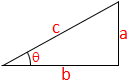# Arcsin Calculator

In mathematics, the inverse trigonometric functions are the inverse functions of the trigonometric functions. Specifically, the arcsin is the inverse of the sine. It is normally represented by arcsin(θ) or sin-1(θ).arcsin = ?